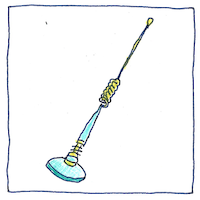## Heaviside condition

In a transmission line, when the ratio of conductance over capacitance equals the ratio of resistance over inductance, when the ratio of siemens per meter over farads per meter equals the ratio of ohms per meter over henries per meter, then a transmission will not be distorted.

## Lenz’s law

Heinrich Friedrich Lenz explained that a changing magnetic field in a conductor induces a magnetic field in the opposite direction which is why in a circuit an inductor opposes an alternating current. This is called inductive reactance.

## Intuitive degree

At some point, I assume, if I study and experiment, then the laws of electromagnetism will become intuitive. I’ll see clearly the relations between conductance and resistance, capacitance and inductance, and see how the tiny components work, but now I struggle to comprehend. Until then, I’ll feel deprived, like a person who knows what is beautiful but cannot feel its joy or pain.

An inductor with an inductance of 1 henry produces an electromotive force of 1 volt when the current through the inductor changes at the rate of 1 ampere per second.